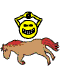## AOS Swing Speed Increase prop.

Here you can post threads on the development of the current release of the core (100)

Moderator: POL Developer

DevGIB
Grandmaster Poster
Posts: 244
Joined: Mon Feb 06, 2006 6:12 am

### AOS Swing Speed Increase prop.

Now that the other non-invasive AOS properties added i'm looking at some of the ones that were skipped due to the link with other core internal calculations.

The first consideration is Swing Speed Increase.

Consider the following:
Currently a weapon can have either a 'Delay' or 'Speed' value set.
Working with the Speed value (as i think this is how most servers use it).
If we had a weapon with a value of 30.
Lets say through mods on other items and the player itself they have a total calculated swing_speed_increase value of 100.

How would this effect the actual speed of the weapon?

A) Its used as a percentage calculator additional to the root speed of the weapon.
e.g. Speed is 30, swing_speed_Increase is 100, 100% of 30 is 30, so speed value is 30+30=60.
If the swing_speed_increase was 90
90% of 30 is 27, root value 30+27 = calculated speed value of 57

B) Its used as a direct modifier to the speed value.
e.g. Speed is 30, swing_speed_increase is 100, calculated speed value is 130.

C) No core calculation is done, speed is left as it is, developers are encouraged to use the swing_speed_increase in either hit scripts or combat hooks.

Similar considerations would need to be made for damage increase, but 1 hurdle at a time.

Discuss.

guialtran
Grandmaster Poster
Posts: 120
Joined: Wed Jul 30, 2008 12:42 pm

### Re: AOS Swing Speed Increase prop.

observing the speed and delay point of view
I think I would leave this variable only for access to the status interface.
which allows the programmer to create any range 0-5, 0-100, 0-1000 and internally there is a calculation of unseen speed gain, or decrease in delay.